• python浮点数
千次阅读
2022-03-18 09:12:54

0b或0B 二进制0，1

0o或0O 八进制0，1，2，3，4，5，6，7

0x或0X 十六进制0，1，2，3，4，5，6，7，8，9，A,B,C,D,E,F

使用int（）实现类型转换：

1.浮点数直接舍去小数部分，例如int(8.9)=8

2.布尔型，true为1,false为0

3.整数和浮点数混合运算，直接转换为浮点型，例如4.0+6=10.0

4.googel大小为10^100

浮点数用科学计数法表示，比如3.14可表示为314e-2或314E-2

float也可将其它类型数转化为浮点数，比如float(3)=3.0

转化字符串，比如float("3.14")=3.14

四舍五入可用round(value)，比如round(3.6)=4

增强型赋值运算符

+=，-=，*=，/=，//=，**=，%=

更多相关内容
• ## Python浮点数的比较

千次阅读 2022-04-04 14:45:35
0.1 + 0.2 不等于 0.3？浮点数改如何进行比较？

目录

问题

原因

解决

参考

# 问题

你是否遇到过这样的问题：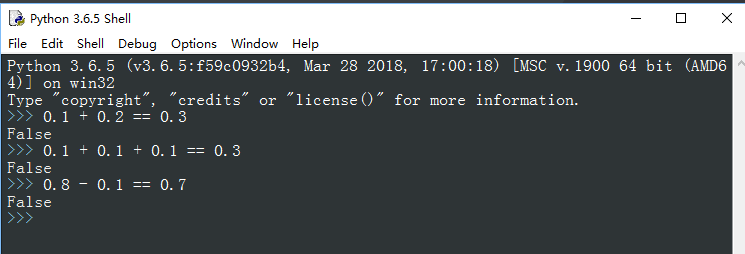然后你开始怀疑人生，究竟是数学老师骗了我，还是计算机骗了我。

答案是，没人骗你，甚至你的数学老师都不曾记得你。

# 原因

之所以出现这种让人摸不着头脑的问题，是因为人类使用数字的习惯和计算机存储处理数字的方式不同。

“0.1”是十进制数字，计算机存储浮点数要将十进制转为二进制。而在十进制位数有限的数字，转为二进制时，就未必还是有限数字。

同时，因为计算机的存储空间有限，对于无限数字只能近似存储，也就出现了开头的问题。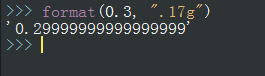# 解决

那该如何避免浮点数进行比较时出现这种状况呢？答案是，不要比较。如何避免买到假货，答案是不要买。这话说的不讲道理，但却无法反驳。

其实说不要比较，是不要用等号去比较，可以换种方式：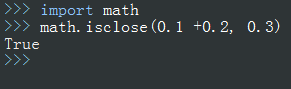isclose函数会根据数字大小，计算一个relative tolerance（相对误差，博主译，未必准确，请自行理解），如果二者之间的距离（差的绝对值）小于relative tolerance，则返回结果为True。

你也可以自定义相对误差数值：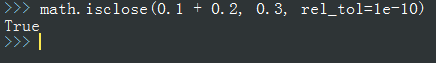之后，需要用等号比较浮点数的地方，都可以用isclose替代。

当然还有其他替代方式，比如numpy中的allclose等等。这些方式虽然都是近似，但在非精密计算下，完全可以满足我们的需求。

# 参考

The Right Way to Compare Floats in Python （收录于Pycoder's Weekly #518 March 29, 2022）

展开全文• python 浮点数计算之---------------- 减法 class float_subtraction(): def __init__(self, _sbt, _mnd): self._mnd = _mnd self._sbt = _sbt self.b_sbt = _sbt if _sbt > _mnd else _mnd self.s_mnd = _...

## python 浮点数计算之 加减乘除

##### ------------------- 适用正负数整数浮点数
class float_subtraction():
def __init__(self, _sbt, _mnd):
self.s_decimal = str(_sbt).split('.')
self.m_decimal = str(_mnd).split('.')
self.obj_change()

def obj_change(self):
if len(self.s_decimal) > 1 and len(self.m_decimal) > 1:
if len(self.s_decimal) > len(self.m_decimal):
self.num = len(self.s_decimal)
else:
self.num = len(self.m_decimal)
elif len(self.s_decimal) > 1 and len(self.m_decimal) == 1:
self.num = len(self.s_decimal)
elif len(self.m_decimal) > 1 and len(self.s_decimal) == 1:
self.num = len(self.m_decimal)
else:
self.num = 0

self.integer = 10 ** self.num
self.s_d = int(self.s_decimal + self.s_decimal + '0' * (self.num - len(self.s_decimal)))
self.m_d = int(self.m_decimal + self.m_decimal + '0' * (self.num - len(self.m_decimal)))

def subtraction(self):
res = (self.s_d - self.m_d) / self.integer
print(res)
return res

res = (self.s_d + self.m_d) / self.integer
print(res)
return res

def multiplication(self):
res = ((self.s_d) * (self.m_d)) / self.integer ** 2
print(res)
return res

def division(self):
res = ((self.s_d) / (self.m_d))
print(res)
return res
if __name__ == '__main__':
c2 = float_subtraction(-8.21, -2.2).addition()
c3 = float_subtraction(-8.21, 2.2).subtraction()
c = float_subtraction(-8.21, -2.2).multiplication()
c1 = float_subtraction(8.21, -2.2).division()


#### 结果展示

-10.41
-10.41
18.062
-3.731818181818182

展开全文python
• ## python浮点数类型

千次阅读 2021-01-11 15:06:16
浮点数间运算存在不确定尾数，这不是bug # 浮点数间运算存在不确定尾数 >>> 0.1+0.2 0.30000000000000004 >>> 0.1+0.3 0.4 >>> 所以，浮点数间比较要使用 round 函数 >>&...

### 浮点数间运算存在不确定尾数，这不是bug

# 浮点数间运算存在不确定尾数
>>> 0.1+0.2
0.30000000000000004
>>> 0.1+0.3
0.4
>>>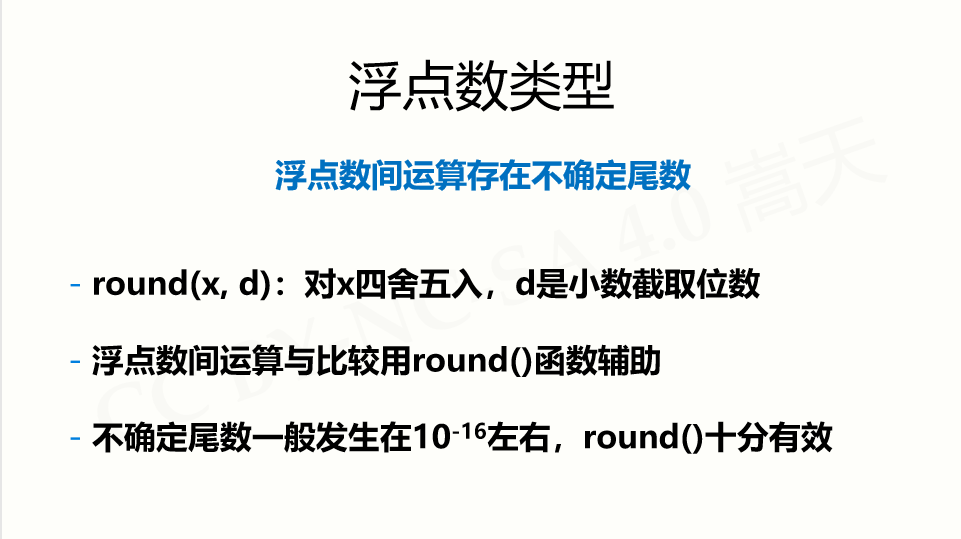>>> 0.1+0.2 == 0.3
False
>>> round(0.1+0.2, 1) == 0.3
True
>>> 

### 数值运算操作符：+、-、*、/、//、%、**

>>> 1+2
3
>>> 1-2
-1
>>> 3*4
12
>>> 3/4
0.75
>>> #  // 表示求商数
>>> 3//4 # 3//4 商0余1,结果是 0
0
>>> 4//3 # 4//3 商1余1,结果是 1
1
>>> 1%3
1
>>> #  ** 表示x的y次方，当 y 是小数的时候，就是开方了
>>> 3**4
81
>>> 9**0.5
3.0
>>> 

### 数值运算函数：

>>> # 绝对值
>>> abs(-10.01)
10.01
>>> # 商余， 同事输出商和余数
>>> divmod(10,3)
(3, 1)
>>> # pow(x, y [,z]) 等价于 (x**y)%z,  x**y 表示x的y次方
>>> pow(3,3)
27
>>> pow(3,3,4) # 表示求3的3次方被4除的余数 27 % 4 = 3
3
>>> # round(x [,d]) 四舍五入，保留 d 位小数
>>> round(-10.123)
-10
>>> round(-10.123, 2)
-10.12
>>> round(10.125, 2)
10.12
>>> # max 取最大值
>>> max(1,9,5,4,6,3)
9
>>> # min 取最小值
>>> min(1,9,5,4,6,3)
1
>>> # int(x),将x变成整数，舍弃小数部分
>>> int(123.45)
123
>>> int("123")
123
>>> # float(x),将x变为浮点数，增加小数部分
>>> float(12) # 结果为12.0
12.0
>>> float("12.34") # 结果为 12.34
12.34
>>>
>>> # complex(x),将x变为复数，增加虚数部分
>>> complex(4)
(4+0j)

展开全文python 浮点数
• ## Python浮点数运算

千次阅读 2020-12-23 16:20:30
在深入了解浮点数的实现之前，先来看几个 Python 浮点数计算有意思的例子：0.1 == 0.10000000000000000000001True0.1+0.1+0.1 == 0.3FalseIEEE 浮点数表示法这些看起来违反常识的“错误”并非 Python 的错，而是由...
• Python浮点数数组求和结果小数点溢出/有误差/小数点不精确的问题。python
• 今天遇到有人问我哥问题，a = 7.1sum= a / 1000print(sum)...其实这不是Python的问题，而是实数的无限精度跟计算机的有限内存之间的矛盾。我们知道，将一个小数转化为二进制表示的方式是，不断的乘2，取其中的整数...
• 我们都知道计算机最底层是二...这是二进制浮点数的自然性质：它不是 Python 中的一个 bug，也不是开发者的代码中的 bug。最后我们要说，虽然对于不同场景下浮点数的处理“没有统一的答案”，但也不要过分惧怕浮点数python
• int1 = 7 float1 = 7.5 trans_int = int(float1) trans_float = int(int1) print(trans_int) # 7 print(trans_float) # 7.0python
• float类型存放双精度的浮点数，具体取值范围依赖于构建python的c编译器，由于精度受限，进行相等性比较不可靠。如果需要高精度，可使用decimal模块的decimal.Decimal，这种类型可以准确的表示循环小数，但是处理速度...
• ## Python浮点数相加

千次阅读 2020-09-19 01:48:47
安装 pip3 install PyDecimal from decimal import Decimal a=0.1 b=0.2 print(Decimal(str(a))+Decimal(str(b))) # 0.3
• 浮点数一个普遍的问题就是在计算机的世界中，浮点数并不能准确地表示十进制。并且，即便是最简单的数学运算，也会带来不可控制的后果。因为，在计算机的世界中只认识0与1。 # 浮点数 print("0.1 + 0.1 = ", 0.1 + ...
• Python使用decimal模块解决浮点数减法精度问题python
• W WW.002pc .COM对《python 浮点数 转 整数python函数每日一讲 - all()》总结来说，为我们python培训很实用。all(iterable)版本：该函数在python2.5版本首次出现，适用于2.5以上版本，包括python3，兼容python3版本...
• Python浮点数高精度表达方法 文章目录 系列文章目录 前言 一、常见的问题 二、解决方案 1.整数运算 2.decimal库 总结 前言 在python浮点数处理过程中，我们常常发现明明使用round方法设置了所需...python
• python中对于一些浮点数的运算结果，往往出现一些意想不到的结果，该博客主要讲解导致这样的原因以及解决办法。python 浮点运算
• 浮点数简单来说就是实数，也叫小数，不同于整数，其带有小数部分。浮点数广泛用于统计和科学计算上。现实生活中，我们经常说的一个半小时，其应该用 1.5 个小时来表示。所有浮点数自然存在于生活中，并且广泛存在...
• 浮点数在计算机硬件中表示为以 2 为基数（二进制）的小数。 举例而言，十进制的小数 0.125=1/10 + 2/100 + 5/1000 同理二进制小数 0.001 0*1/2+0*1/4+1*1/8得十进制的0.125（二进制的小数转换为十进制...
• 本文主要分享基于python的数据分析三方库pandas，numpy的一次爬坑经历，发现并分析了python语言对于浮点数精度处理不准确的问题，并在最后给出合理的解决方案。如果你也在用python处理数据，建议看一下，毕竟0.1的...
• Python的一些长效任务中，不可避免的需要向文本文件、二进制文件或者数据库中写入一些数据，或者是在屏幕上输出一些文本，此时如何控制输出数据的长度是需要我们注意的一个问题。比如对于一个二进制文件，如果输出...python java 人工智能 大数据 机器学习
• 输出的数字和源数据总是有差距如何处理，numpy显示也不好用python
• 0.1 + 0.2 == 0.3 ？ Python 浮点数详解python
• python基础语法.jpg您的“关注”和“点赞...image.png目录Python 浮点数数据类型概述2.2.1 指数表示形式概述4.1 微信公众号：码农阿杰5.1 Python 3.8.2 documentation1 Python 浮点数数据类型概述浮点数数据类型，可...
• 1、浮点数计算出错 a=1.1 ...而python是以双精度(64)位来保存浮点数，多余的位会被截掉，所以看到的是0.1，但在电脑上实际保存的已不是精确的0.1。 【注意】 这是二进制浮点数的自然性质：它不是 Pytpython 浮点数 精度 decimal
• 1 Python 浮点数数据类型概述浮点数数据类型，可以简称为浮点类型。小数通常以浮点数的形式存储，在 Python 中用 float 表示。可以理解为浮点数是用来描述小数的。浮点数通常使用 C 中的 double 来实现。Python 中......